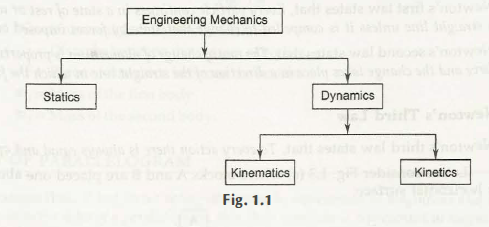Mechanical Engineering is the part of Engineering or Physical Science, in which motion of a body explains about its behavior whether it is at rest or in motion. Both cases are completely different than each other. Mechanical disturbances are always important to know the action on a body. A students need to know that in which condition of body acceleration takes place or at which time it is not constant.

There are two different categories in which Mechanics are divided –

• Statics

The study that deals with the behavior of rest is known as statics. In this case no proper effect of force work and thus it is known as a rigid body. The analysis done on this explains that how loads act on a system which is unable to experience any acceleration. It means its acceleration is 0.

• Dynamics

The study that deals with the motion of a body is known as Dynamics. Dynamics cover a large area in the study where the behavior of matters can be decided by

It is important to know about these motions. In addition, a body at rest means no motion is there and thus statics does not have any type.

Dynamics are available in two different types –

• Kinematics

Kinematics deals with the study of those bodies which do not considering forces. What does this mean? It means when the velocity is constant in a body or you can say that acceleration is 0 or the body moves without any change in acceleration, then it is said as kinematics.

• Kinetics –

The study of a body which is in motion and in which force is considerable is known as Kinetics. It means a change in velocity and acceleration takes place. The causes of motion due to force and also due to torque can be pointed out here.Through a block diagram (above) students can easily understand Engineering Mechanics.

Links of Next Mechanical Engineering Topics:-### Customer Reviews

My Homework Help
Rated 5.0 out of 5 based on 510 customer reviews at